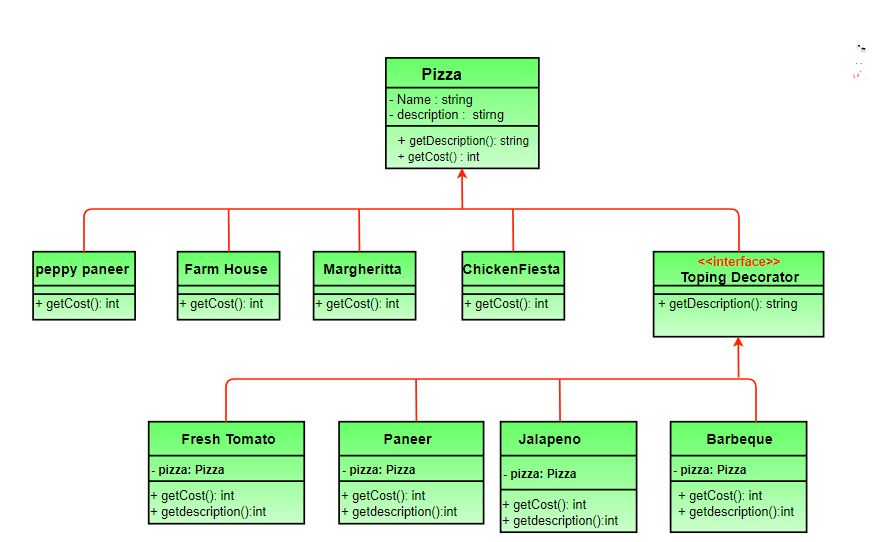GFG App
Open AppBrowser
Continue

# Decorator Pattern | Set 3 (Coding the Design)

We have discussed Pizza design problem and different naive approaches to solve it in set 1. We have also introduced Decorator pattern in Set 2. In this article, design and implementation of decorator pattern for Pizza problem is discussed. It is highly recommended that you try it yourself first. The new class diagram (Click on the picture to see it clearly)• Pizza acts as our abstract component class.
• There are four concrete components namely PeppyPaneer , FarmHouse, Margherita, ChickenFiesta.
• ToppingsDecoratoris our abstract decorator and FreshTomato , Paneer, Jalapeno, Barbeque are concrete decorators.

Below is the java implementation of above design.

## Java

 `// Java program to demonstrate Decorator` `// pattern`   `// Abstract Pizza class (All classes extend` `// from this)` `abstract` `class` `Pizza` `{` `    ``// it is an abstract pizza` `    ``String description = "Unkknown Pizza";`   `    ``public` `String getDescription()` `    ``{` `        ``return` `description;` `    ``}`   `    ``public` `abstract` `int` `getCost();` `}`   `// The decorator class :  It extends Pizza to be` `// interchangeable with it toppings decorator can` `// also be implemented as an interface` `abstract` `class` `ToppingsDecorator ``extends` `Pizza` `{` `    ``public` `abstract` `String getDescription();` `}`   `// Concrete pizza classes` `class` `PeppyPaneer ``extends` `Pizza` `{` `    ``public` `PeppyPaneer() { description = "PeppyPaneer"; }` `    ``public` `int` `getCost() {  ``return` `100``; }` `}` `class` `FarmHouse ``extends` `Pizza` `{` `    ``public` `FarmHouse() {  description = "FarmHouse"; }` `    ``public` `int` `getCost() { ``return` `200``; }` `}` `class` `Margherita ``extends` `Pizza` `{` `    ``public` `Margherita()  { description = "Margherita"; }` `    ``public` `int` `getCost() { ``return` `100``;  }` `}` `class` `ChickenFiesta ``extends` `Pizza` `{` `    ``public` `ChickenFiesta() { description = "ChickenFiesta";}` `    ``public` `int` `getCost() { ``return` `200``; }` `}` `class` `SimplePizza ``extends` `Pizza` `{` `public` `SimplePizza() { description = "SimplePizza"; }` `public` `int` `getCost() {  ``return` `50``;  }` `}`   `// Concrete toppings classes` `class` `FreshTomato ``extends` `ToppingsDecorator` `{` `    ``// we need a reference to obj we are decorating` `    ``Pizza pizza;`   `    ``public` `FreshTomato(Pizza pizza) { ``this``.pizza = pizza; }` `    ``public` `String getDescription() {` `        ``return` `pizza.getDescription() + ", Fresh Tomato ";` `    ``}` `    ``public` `int` `getCost() { ``return` `40` `+ pizza.getCost(); }` `}` `class` `Barbeque ``extends` `ToppingsDecorator` `{` `    ``Pizza pizza;` `    ``public` `Barbeque(Pizza pizza) {  ``this``.pizza = pizza;  }` `    ``public` `String getDescription() {` `        ``return` `pizza.getDescription() + ", Barbeque ";` `    ``}` `    ``public` `int` `getCost() { ``return` `90` `+ pizza.getCost(); }` `}` `class` `Paneer ``extends` `ToppingsDecorator` `{` `    ``Pizza pizza;` `    ``public` `Paneer(Pizza pizza)  {  ``this``.pizza = pizza; }` `    ``public` `String getDescription() {` `        ``return` `pizza.getDescription() + ", Paneer ";` `    ``}` `    ``public` `int` `getCost()  {  ``return` `70` `+ pizza.getCost(); }` `}`   `// Other toppings can be coded in a similar way`   `// Driver class and method` `class` `PizzaStore` `{` `    ``public` `static` `void` `main(String args[])` `    ``{` `        ``// create new margherita pizza` `        ``Pizza pizza = ``new` `Margherita();` `        ``System.out.println( pizza.getDescription() +` `                         ``" Cost :" + pizza.getCost());`   `        ``// create new FarmHouse pizza` `        ``Pizza pizza2 = ``new` `FarmHouse();`   `        ``// decorate it with freshtomato topping` `        ``pizza2 = ``new` `FreshTomato(pizza2);`   `        ``//decorate it with paneer topping` `        ``pizza2 = ``new` `Paneer(pizza2);`   `        ``System.out.println( pizza2.getDescription() +` `                         ``" Cost :" + pizza2.getCost());` `        ``Pizza pizza3 = ``new` `Barbeque(``null``);    ``//no specific pizza` `        ``System.out.println( pizza3.getDescription() + "  Cost :" + pizza3.getCost());` `   ``}` `}`

Output:

```Margherita Cost :100
FarmHouse, Fresh Tomato , Paneer Cost :310```

Notice how we can add/remove new pizzas and toppings with no alteration in previously tested code and toppings and pizzas are decoupled.

## CPP

 `// CPP program to demonstrate ` `// Decorator pattern` `#include ` `#include ` `using` `namespace` `std;`   `// Component ` `class` `MilkShake` `{` `public``:` `    ``virtual` `string Serve() = 0;` `    ``virtual` `float` `price() = 0;` `};`     `// Concrete Component  ` `class` `BaseMilkShake : ``public` `MilkShake` `{` `public``:` `    ``string Serve()` `    ``{` `        ``return` `"MilkShake";` `    ``}`   `    ``float` `price()` `    ``{` `        ``return` `30;` `    ``}` `};`   `// Decorator ` `class` `MilkShakeDecorator: ``public` `MilkShake` `{` `protected``:` `    ``MilkShake *m_MilkShake;` `public``:`   `    ``MilkShakeDecorator(MilkShake *baseMilkShake): m_MilkShake(baseMilkShake){}`   `    ``string Serve()` `    ``{` `        ``return` `m_MilkShake->Serve();` `    ``}`   `    ``float` `price()` `    ``{` `        ``return` `m_MilkShake->price();` `    ``}` `};`     `// Concrete Decorator ` `class` `MangoMilkShake: ``public` `MilkShakeDecorator` `{` `public``:` `    ``MangoMilkShake(MilkShake *baseMilkShake): MilkShakeDecorator(baseMilkShake){}`   `    ``string Serve()` `    ``{` `        ``return` `m_MilkShake->Serve() + " decorated with Mango ";` `    ``}` `    ``float` `price()` `    ``{` `        ``return` `m_MilkShake->price() + 40;` `    ``}` `};`     `class` `VanillaMilkShake: ``public` `MilkShakeDecorator` `{` `public``:` `    ``VanillaMilkShake(MilkShake *baseMilkShake): MilkShakeDecorator(baseMilkShake){}`   `    ``string Serve()` `    ``{` `        ``return` `m_MilkShake->Serve() + " decorated with Vanilla ";` `    ``}` `    ``float` `price()` `    ``{` `        ``return` `m_MilkShake->price() + 80;` `    ``}` `};`   `int` `main()` `{` `  ``MilkShake *baseMilkShake = ``new` `BaseMilkShake();` `  ``cout << "Basic Milk shake \n";` `  ``cout << baseMilkShake -> Serve() << endl;` `  ``cout << baseMilkShake -> price() << endl;    `   `  ``MilkShake *decoratedMilkShake = ``new` `MangoMilkShake(baseMilkShake);` `  ``cout << "Mango decorated Milk shake \n";` `  ``cout << decoratedMilkShake -> Serve() << endl;` `  ``cout << decoratedMilkShake -> price() << endl;    ` `    `  `  ``delete` `decoratedMilkShake;`   `  ``decoratedMilkShake = ``new` `VanillaMilkShake(baseMilkShake);` `  ``cout << "Vanilla decorated Milk shake \n";` `  ``cout << decoratedMilkShake -> Serve() << endl;` `  ``cout << decoratedMilkShake -> price() << endl;    `   ` ``delete` `decoratedMilkShake;` ` ``delete` `baseMilkShake;` `  ``return` `0;` `} `

Output:

```Basic Milk shake
MilkShake
Price of MilkShake : 30
Mango decorated Milk shake
MilkShake decorated with Mango
Price of Mango MilkShake : 70
Vanilla decorated Milk shake
MilkShake decorated with Vanilla
Price of Vanilla MilkShake : 110```

Further Read: Decorator Method in Python

This article is contributed by Sulabh Kumar and Shashank Gupta. If you like GeeksforGeeks and would like to contribute, you can also write an article and mail your article to review-team@geeksforgeeks.org. See your article appearing on the GeeksforGeeks main page and help other Geeks.